# JP3783695B2 - Motor control device - Google Patents

## Info

Publication number
JP3783695B2
JP3783695B2 JP2003078181A JP2003078181A JP3783695B2 JP 3783695 B2 JP3783695 B2 JP 3783695B2 JP 2003078181 A JP2003078181 A JP 2003078181A JP 2003078181 A JP2003078181 A JP 2003078181A JP 3783695 B2 JP3783695 B2 JP 3783695B2
Authority
JP
Japan
Prior art keywords
motor
phase
axis current
axis
voltage
Prior art date
Legal status (The legal status is an assumption and is not a legal conclusion. Google has not performed a legal analysis and makes no representation as to the accuracy of the status listed.)
Active
Application number
JP2003078181A
Other languages
Japanese (ja)
Other versions
JP2004289926A (en
Inventor

Original Assignee

Priority date (The priority date is an assumption and is not a legal conclusion. Google has not performed a legal analysis and makes no representation as to the accuracy of the date listed.)
Filing date
Publication date
Application filed by 日産自動車株式会社 filed Critical 日産自動車株式会社
Priority to JP2003078181A priority Critical patent/JP3783695B2/en
Publication of JP2004289926A publication Critical patent/JP2004289926A/en
Application granted granted Critical
Publication of JP3783695B2 publication Critical patent/JP3783695B2/en
Active legal-status Critical Current
Anticipated expiration legal-status Critical

• 238000006243 chemical reaction Methods 0.000 claims description 16
• 230000001360 synchronised Effects 0.000 claims description 10
• 238000004364 calculation methods Methods 0.000 claims description 5
• 230000004044 response Effects 0.000 description 4
• 230000000694 effects Effects 0.000 description 3
• 230000001276 controlling effects Effects 0.000 description 2

## Images

•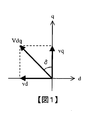•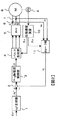•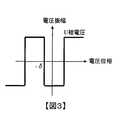•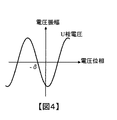•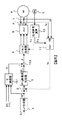•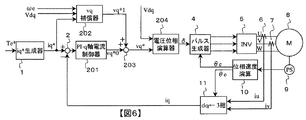•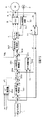•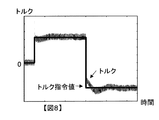## Classifications

• HELECTRICITY
• H02GENERATION; CONVERSION OR DISTRIBUTION OF ELECTRIC POWER
• H02PCONTROL OR REGULATION OF ELECTRIC MOTORS, ELECTRIC GENERATORS OR DYNAMO-ELECTRIC CONVERTERS; CONTROLLING TRANSFORMERS, REACTORS OR CHOKE COILS
• H02P25/00Arrangements or methods for the control of AC motors characterised by the kind of AC motor or by structural details
• H02P25/02Arrangements or methods for the control of AC motors characterised by the kind of AC motor or by structural details characterised by the kind of motor
• H02P25/022Synchronous motors
• H02P25/024Synchronous motors controlled by supply frequency
• HELECTRICITY
• H02GENERATION; CONVERSION OR DISTRIBUTION OF ELECTRIC POWER
• H02PCONTROL OR REGULATION OF ELECTRIC MOTORS, ELECTRIC GENERATORS OR DYNAMO-ELECTRIC CONVERTERS; CONTROLLING TRANSFORMERS, REACTORS OR CHOKE COILS
• H02P21/00Arrangements or methods for the control of electric machines by vector control, e.g. by control of field orientation
• H02P21/22Current control, e.g. using a current control loop

## Description


BACKGROUND OF THE INVENTION
The present invention relates to an AC motor control device.

[Prior art]
When applying voltage to an AC motor using an inverter, applying a pulse of rectangular wave voltage instead of sinusoidal PWM voltage can increase the peak value of the fundamental wave voltage, and the motor output in the high rotation speed range. Can be increased. During such rectangular wave voltage driving, the phase of the voltage applied to the motor can be controlled, but the amplitude of the applied voltage is determined by the DC power supply voltage (DC link voltage) of the inverter, and the motor torque cannot be controlled accurately.

In order to solve such a problem at the time of rectangular wave voltage driving, a torque estimator is used to estimate the motor torque, and by controlling the voltage phase based on the deviation between the torque command value and the estimated value, the torque command 2. Description of the Related Art A motor control device that can obtain a torque corresponding to a value is known (see, for example, Patent Document 1).

Prior art documents related to the invention of this application include the following.
[Patent Document 1]
Japanese Patent Laid-Open No. 2000-050689
[Problems to be solved by the invention]
However, in the above-described conventional motor control device, the estimated value is fed back to the command value of the motor torque based on the relationship between the motor torque and the voltage phase in the steady state, and thus the torque control is performed. It is difficult to increase responsiveness. In addition, since the torque estimator estimates the motor torque from the relationship between the electric power, the machine output, and the loss, it is difficult to improve the torque control accuracy because an accurate estimated value cannot be obtained.

The present invention provides a motor control device with improved torque control performance when a synchronous motor is driven by a rectangular wave voltage.

[Means for Solving the Problems]
In the present invention, the current flowing through the three-phase synchronous motor is coordinate-converted into a dq-axis current in a dq-axis coordinate system that rotates in synchronization with the motor rotation, and based on a q-axis current deviation between the q-axis current command value and the q-axis current. The phase of the rectangular wave voltage is calculated, and a rectangular wave voltage is generated from the DC power source according to this phase and applied to the three-phase synchronous motor for driving.

【The invention's effect】
According to the present invention, it is possible to improve torque control performance during rectangular wave voltage driving.

DETAILED DESCRIPTION OF THE INVENTION
The circuit equation in the dq axis coordinate system rotating in synchronization with the rotation of the permanent magnet synchronous motor can be expressed as the following equation.
[Expression 1]
In Equation 1, vd is a d-axis voltage, vq is a q-axis voltage, Ld is a d-axis inductance, Lq is a q-axis inductance, R is an armature resistance, ωe is an electric angular velocity of the motor, and id is a field field of the motor. The d-axis current corresponding to the current, iq is the q-axis current corresponding to the torque current of the motor, φ is the number of flux linkages by the permanent magnet, and p is the differential operator.

Considering a steady state in which the motor load, that is, the current is substantially constant, Equation 1 can be approximated as follows.
[Expression 2]
Further, when the motor is rotating at high speed, the influence of the armature resistance R and the voltage due to the dq-axis currents id and iq is smaller than the voltage due to the dq-axis inductances Ld and Lq and the dq-axis currents id and iq. Equation 2 can be further approximated as the following equation.
[Equation 3]

Here, when vd and vq are expressed using the rectangular wave voltage phase δ, the relationship is expressed by the following equation, and the relationship shown in FIG. 1 is illustrated.
[Expression 4]
vd = -Vdq · sinδ
[Equation 5]
vq = Vdq ・ cosδ
In Equations 4 and 5, Vdq is the magnitude of the voltage vector in the dq axis coordinate system (hereinafter simply referred to as the dq axis voltage), and when the rectangular wave voltage is driven, the DC power supply voltage (DC link voltage) Vdc of the inverter is used. It can be expressed as:
[Formula 6]
Vdq = √ (6) · Vdc / π
From Equation 3 and Equation 4, the following equation is derived.
[Expression 7]
iq = Vdq · sinδ / Lqωe
From Equation 7, it is understood that the q-axis current iq changes in a sinusoidal shape with respect to the voltage phase δ in a steady state at a high rotational speed. Further, in the range of δ = −π / 2 to π / 2, iq monotonically increases with respect to δ, and therefore, the q-axis current that is a torque component current can be controlled by operating δ.

Therefore, in the following, an embodiment in which the motor torque is adjusted by operating the voltage phase δ to control the q-axis current iq, which is a torque-divided current, as described with Expression 7 will be described.

<< First Embodiment of the Invention >>
FIG. 2 shows the configuration of the first embodiment. The iq * generator 1 receives the torque command value Te * of the synchronous motor 8 and generates a q-axis current command value iq * corresponding to the torque command value Te * . This iq * generator is constituted by a map of iq * using, for example, torque and speed as variables. The phase speed calculator 10 calculates the electrical rotation speed ωe and the electrical rotation angle θe of the motor 8 based on the mechanical rotation angle of the motor 8 detected by the position sensor 9 such as a resolver. The dq ← three-phase converter 11 converts the three-phase alternating currents iu and iv detected by the current sensors 6 and 7 using the electrical rotation angle θe of the motor 8 by the following equation to obtain the q-axis current iq. .
[Equation 8]
The W-phase AC current iw may be detected by a current sensor in the same manner as the U and V phases, but can be calculated by the following equation from the relationship of the three-phase AC.
[Equation 9]
iw = -iu-iv

The subtractor 2 and the PI-q axis current controller 3 perform feedback control of the q axis current iq. First, the subtractor 9 calculates a deviation (iq * −iq) between the q-axis current command value iq * and the q-axis current iq. Next, the PI-q-axis current controller 3 performs PI control (proportional P / integral I control) so that the q-axis current deviation (iq * -iq) becomes 0 to obtain the phase δ of the rectangular wave voltage. . The q-axis current controller may be a PID controller, and the rectangular wave voltage phase δ may be calculated by performing PID control on the q-axis current deviation. When the rectangular wave voltage is driven, the phase δ of the rectangular wave voltage applied to the motor 8 has the relationship shown in FIG. When the sine wave PWM voltage is driven, the phase δ of the sine wave PWM voltage applied to the motor 8 has the relationship shown in FIG.

The pulse generator 4 generates a three-phase rectangular wave voltage command to be applied to the motor 8 based on the rectangular wave voltage phase δ and the electrical rotation angle θe of the motor 8. Here, in normal vector control in which the d-axis current and the q-axis current are controlled independently, coordinate conversion from the dq-axis voltages vd and vq to the three-phase AC voltages vu, vv and vw is performed according to the following equation.
[Expression 10]
Substituting vd and vq shown in equations 4 and 5 into dq-axis voltages vd and vq in equation 10 yields the following equation.
## EQU11 ##
Although Equation 11 represents a sine wave voltage, a three-phase rectangular wave voltage corresponding to the sign of the sine wave is output when the rectangular wave voltage is driven.

The inverter 5 drives the switching element in accordance with the rectangular wave voltage command generated by the pulse generator 4, generates a three-phase rectangular wave voltage of phase δ from a DC power supply (DC link), and applies it to the motor 8.

When the inverter is operated with rectangular wave voltage control, a fundamental wave voltage 27.3% higher than that when operated with sine wave PWM voltage control can be applied to the motor. In addition, a method of improving the voltage utilization factor by superimposing a third harmonic on a sine wave is known, but a fundamental wave voltage that is 10.3% higher than this method when operated by rectangular wave voltage control. Can be applied to the motor.

Thus, according to the first embodiment, PI (or PID) is fed so that the q-axis current deviation (iq * −iq) becomes zero by feeding back the q-axis current iq to the q-axis current command value iq *. ) A rectangular wave voltage phase δ is obtained by performing control, and a three-phase rectangular wave voltage having a phase δ is applied to the motor, so that a higher fundamental wave voltage can be used compared to the sine wave PWM voltage driving of the motor. This increases the output in the high speed region of the motor. Further, the q-axis current that is the torque current can be controlled with high accuracy, and the torque control accuracy when the synchronous motor is driven with the rectangular wave voltage can be improved.

In the first embodiment described above, the current sensors 6 and 7 are the current detection means, the position sensor 9, the phase speed calculator 10 and the dq ← three-phase converter 11 are the current conversion means, the subtractor 2 and the PI−. The q-axis current controller 3 constitutes a phase calculation means, and the pulse generator 4 and the inverter 5 constitute a power conversion means. In addition, as long as the characteristic function of this invention is not impaired, each component is not limited to the said structure.

<< Second Embodiment of the Invention >>
In the first embodiment, an example in which the q-axis current iq is fed back to the q-axis current command value iq * and PI (or PID) control is performed on the deviation (iq * −iq) has been described. When the period is long, it becomes difficult to set a PI (or PID) control gain (proportional P gain, integral I gain, differential D gain) high, and the responsiveness of the q-axis current control decreases. Therefore, in the second embodiment, a feedforward compensator is added to the first embodiment described above to improve the responsiveness of the q-axis current control.

FIG. 5 shows the configuration of the second embodiment. In addition, the same code | symbol is attached | subjected to the apparatus similar to the apparatus of 1st Embodiment shown in FIG. 2, and description is abbreviate | omitted. The feedforward δ compensator 101 compensates for the rectangular wave voltage phase δo of the PI-q axis current controller 3 based on the electrical angular velocity ωe of the motor 8, the dq axis voltage Vdq, and the q axis current command value iq *. Is obtained. The adder 102 adds the rectangular wave voltage phase δo of the PI-q axis current controller 3 and the rectangular wave voltage phase compensation δ ′ of the δ compensator 101 to obtain the rectangular wave voltage phase δ. The configuration other than the δ compensator 101 and the adder 102 is the same as the configuration of the first embodiment shown in FIG.

The configuration of the δ compensator 101 will be described in more detail. In the relationship between the rectangular wave voltage phase δ, the dq axis voltage Vdq, the electrical angular velocity ωe of the motor 8 and the q axis current iq shown in Equation 7, sin δ≈δ is approximated, and the q axis current command value iq * From the target angular velocity ωe and the dq axis voltage Vdq, a compensation amount δ ′ of the rectangular wave voltage phase is obtained by the following equation.
[Expression 12]
δ '= Lqωe · iq * / Vdq
The dq axis voltage Vdq is obtained from the DC power supply voltage (DC link voltage) Vdc of the inverter 5 using the above equation 6.

The subtractor 2 and the PI-q axis current controller 3 perform the PI (or PID) control so that the q axis current deviation (iq * −iq) becomes 0 as described above to obtain the rectangular wave voltage phase δo. . The adder 102 adds the rectangular wave voltage phase δo of the PI-q axis current controller 3 and the compensation amount δ ′ of the δ compensator 101 to obtain the rectangular wave voltage phase δ. Hereinafter, similarly to the first embodiment, the pulse generator 4 generates a three-phase rectangular wave voltage command to be applied to the motor 8 based on the rectangular wave voltage phase δ and the electrical rotation angle θe of the motor 8, The inverter 5 applies a three-phase rectangular wave voltage of phase δ to the motor 8 to drive it.

As described above, according to the second embodiment, PI (or PID) is fed so that the q-axis current deviation (iq * −iq) becomes zero by feeding back the q-axis current iq to the q-axis current command value iq *. ) To obtain the rectangular wave voltage phase δo by performing control, and the feedforward δ compensator 101 compensates for the rectangular wave voltage phase based on the q-axis current command value iq * , the electrical angular velocity ωe of the motor 8 and the dq-axis voltage Vdq. The minute δ ′ is obtained, and the rectangular wave voltage phase δ is obtained by adding the compensation amount δ ′ to the rectangular wave voltage phase δo. Since the three-phase rectangular wave voltage of phase δ is generated and applied to the motor 8, in addition to the effect of the first embodiment described above, the response of the q-axis current control even when the control period is long. Can be improved.

In the second embodiment described above, the current sensors 6 and 7 are the current detection means, the position sensor 9, the phase speed calculator 10 and the dq ← three-phase converter 11 are the current conversion means, the subtractor 2 and the PI−. The q-axis current controller 3 is the phase calculation means, the pulse generator 4 and the inverter 5 are the power conversion means, the position sensor 9 and the phase speed calculator 10 are the speed detection means, and the δ compensator 101 and the adder 102 are the phase. Compensation means are respectively configured. In addition, as long as the characteristic function of this invention is not impaired, each component is not limited to the said structure.

<< Third Embodiment of the Invention >>
FIG. 6 shows the configuration of the third embodiment. 2 and 5 are denoted by the same reference numerals and description thereof is omitted.

In the third embodiment, PI (or PID) control is performed by the PI-q-axis current controller 201 so that the q-axis current deviation (iq * -iq) becomes 0, and the q-axis voltage vqo is obtained. The q-axis voltage compensation value vq ′ is obtained by the feedforward vq compensator 202 based on the q-axis current command value iq * , the electrical angular velocity ωe of the motor 8 and the dq-axis voltage Vdq, and the q-axis voltage vqo is compensated for vq ′. Is calculated from the q-axis voltage vq and the dq-axis voltage Vdq.

The PI-q-axis current controller 201 performs PI (or PID) control on the deviation (iq * -iq) between the q-axis current command value iq * and the q-axis current iq obtained by the subtracter 2 to obtain the q-axis current. A q-axis voltage command value vq * 0 is determined so that the deviation (iq * −iq) becomes zero. The feedforward vq compensator 202 calculates the q-axis voltage command value vq * 0 of the PI-q-axis current controller 201 based on the q-axis current command value iq * , the electrical angular velocity ωe of the motor 8 and the dq-axis voltage Vdq. A compensation amount vq * 1 for compensation is obtained.

The configuration of the vq compensator 202 will be described in more detail. From the relationship between the d-axis voltage vd, the q-axis voltage vq, and the dq-axis voltage Vdq shown in FIG.
[Formula 13]
vq = √ (Vdq 2 −Lq 2 iq * 2 ωe 2 )
The vq compensator 202 outputs the q-axis voltage vq obtained by Equation 13 as the q-axis voltage command value compensation vq * 1.

The adder 203, PI-q q-axis voltage command value of the axis current controller 201 vq * 0 and vq compensator 202 of the q-axis voltage command value compensation amount vq * 1 and the addition to q-axis voltage command value vq * Ask for. Based on the q-axis voltage command value vq * and the dq-axis voltage Vdq, the voltage phase calculator 204 obtains a rectangular wave voltage phase δ from the relationship shown in FIG.
[Expression 14]
δ = cos −1 (vq * / Vdq)
Hereinafter, similarly to the first embodiment, the pulse generator 4 generates a three-phase rectangular wave voltage command to be applied to the motor 8 based on the rectangular wave voltage phase δ and the electrical rotation angle θe of the motor 8, The inverter 5 applies a three-phase rectangular wave voltage of phase δ to the motor 8 to drive it.

As described above, according to the third embodiment, the q-axis current deviation (iq * −iq) is subjected to PI (or PID) control to obtain the q-axis voltage command value vq * 0 and the feedforward vq compensation. Based on the q-axis current command value iq * , the electric angular velocity ωe of the motor 8 and the dq-axis voltage Vdq, the q-axis voltage command value compensation vq * 1 is obtained by the controller 202 and is compensated for the q-axis voltage command value vq * 0. The q-axis voltage command value vq * is obtained by adding vq * 1. Since the rectangular wave voltage phase δ is obtained from the q-axis voltage command value vq * and the dq-axis voltage Vdq and the three-phase rectangular wave voltage having the phase δ is applied to the motor 8, the first and second described above. In addition to obtaining the same effect as the embodiment, the compensation amount vq * 1 of the q-axis voltage command value vq * 0 is obtained by feedforward control. Therefore, the rotational speed of the motor 8 and the q-axis current command value iq * It is possible to improve the responsiveness of the q-axis current with respect to changes in.

According to the third embodiment, since the rectangular wave voltage phase δ is obtained based on the q-axis voltage vq and Vdq obtained from the DC power supply voltage (DC link voltage) Vdc of the inverter 5, the DC power supply of the inverter 5 is used. Even when the voltage (DC link voltage) Vdc fluctuates, the q-axis current iq can be controlled with good responsiveness, and the responsiveness of torque control is improved.

Furthermore, in the third embodiment, since the PI-q axis current controller 201 can be configured by a controller having the same configuration as that of normal vector control, a change from a motor control device using normal vector control, Improvement is easy.

In the third embodiment, the current sensors 6 and 7 are the current detection means, the position sensor 9, the phase speed calculator 10 and the dq ← three-phase converter 11 are the current conversion means, the subtractor 2, and the PI-q axis. The current controller 201 and the voltage phase calculator 204 are phase calculation means, the pulse generator 4 and the inverter 5 are power conversion means, the position sensor 9 and the phase speed calculator 10 are speed detection means, and the vq compensator 202 and the addition. The units 203 constitute phase compensation means. In addition, as long as the characteristic function of this invention is not impaired, each component is not limited to the said structure.

<< Fourth Embodiment of the Invention >>
As described above, Equation (3) can be obtained by approximating the circuit equation (Equation 1) in the dq axis coordinate system of the permanent magnet synchronous motor at a high rotational speed and limited to a steady state. When Formula 3 is expanded, the following formula is obtained.
[Expression 15]
vd = -Lqωe · iq
[Expression 16]
vq = Ldωe ・ id + ωeφ
That is, as is clear from Equation 15, the q-axis current iq that is the torque current of the motor can be controlled by the d-axis voltage vd at a high rotational speed and in a steady state.

On the other hand, as is clear from Equation 16, the q-axis voltage vq can be reduced by reducing the d-axis current id at a high rotational speed and in a steady state. As is clear from the relationship between the d-axis voltage vd and the q-axis voltage vq shown in FIG. 1, if the q-axis voltage vq is decreased, the d-axis voltage vd can be increased. Increasing the voltage vd increases the q-axis current iq that is the torque current. That is, by reducing the d-axis current id, the q-axis current iq, which is the torque component current, can be increased, thereby improving the torque control accuracy.

In the fourth embodiment, this relationship is used, and in the q-axis current feedback control system, PI (or PID) control in which a negative control gain is set to the q-axis current deviation (iq * −iq) is performed to perform d-axis current. The command value id * is obtained, and then the d-axis current deviation (id * -id) is subjected to PI (or PID) control in the d-axis feedback control system to obtain the d-axis voltage command value vd *. A rectangular wave voltage phase δ is obtained from the value vd * and the dq axis voltage Vdq.

FIG. 7 shows the configuration of the fourth embodiment. In addition, the same code | symbol is attached | subjected to the apparatus similar to the apparatus shown in FIG.2, FIG.5 and FIG.6, and it demonstrates centering on difference. The PI-q-axis current controller 301 multiplies the output obtained by subjecting the q-axis current deviation (iq * -iq) to PI (or PID) control by a gain “−1” and d-axis current command value id *. Find 0.

Further, since only the feedback control of the q-axis current causes a delay in the d-axis current command value id * 0, an id * compensator 302 that is a feedforward compensator is provided to compensate for this delay. The id * compensator 302 calculates a compensation amount id * 1 of the d-axis current command value id * 0 based on the q-axis current command value iq * , the electric rotational speed ωe of the motor 8 and the dq-axis voltage Vdq. From the relationship between the d-axis voltage vd, the q-axis voltage vq, and the dq-axis voltage Vdq shown in FIG.
[Expression 17]
id = 1 / Ld {-φ + √ (Vdq 2 / ωe 2 -Lq 2 iq 2)}
The id * compensator 302 substitutes the command value iq * for the q-axis current iq in Expression 17, and outputs the calculated d-axis current id as the d-axis current command value compensation part id * 1.

The adder 303 adds the d-axis current command value id * 0 of the PI-q-axis current controller 301 and the d-axis current command value compensation part id * 1 of the id * compensator 302 to add the d-axis current command value id. * Ask for.

The subtractor 304 and the PI-d axis current controller 305 perform feedback control of the d axis current id. The d-axis current id is the q-axis current iq and the dq ← three-phase converter 11 and the three-phase AC currents iu and iv detected by the current sensors 6 and 7 by the dq ← three-phase converter 11 and Obtained by coordinate transformation. First, the subtractor 304 calculates a deviation (id * −id) between the d-axis current command value id * and the d-axis current id. Next, the PI-d-axis current controller 305 performs PI (or PID) control so that the d-axis current deviation (id * -id) becomes 0 to obtain the d-axis voltage command value vd * 0.

In the d-axis current control, the voltage generated by the q-axis current works as a disturbance term. Therefore, a non-interference controller 306 is provided to compensate for the disturbance voltage. The non-interference controller 306 obtains the d-axis voltage vd by Equation 15 based on the q-axis current command value iq * and the electrical angular velocity ωe of the motor 8, and uses it as a compensation vd * 1 for the d-axis voltage command value vd * 0. Output. The adder 308 adds the d-axis voltage command value vd * 0 of the PI-d-axis current controller 305 and the d-axis voltage compensation vd * 1 of the non-interference controller 306 to obtain the d-axis voltage command value vd * . .

The voltage phase calculator 307 obtains a rectangular wave voltage phase δ from the relationship shown in FIG. 1 based on the d-axis voltage command value vd * and the dq-axis voltage Vdq by the following equation.
[Formula 18]
δ = sin −1 (−vd * / Vdq)
Hereinafter, similarly to the first embodiment, the pulse generator 4 generates a three-phase rectangular wave voltage command to be applied to the motor 8 based on the rectangular wave voltage phase δ and the electrical rotation angle θe of the motor 8, The inverter 5 applies a three-phase rectangular wave voltage of phase δ to the motor 8 to drive it.

According to the fourth embodiment, the q-axis current iq can be made to follow the command value iq * by controlling the phase δ of the rectangular wave voltage, and the motor torque can be accurately controlled. The responsiveness of the motor torque can be improved.

Further, when the change amount of the d-axis current id with respect to the q-axis current iq is compared with the change amount of the q-axis current iq with respect to the d-axis current id, the latter is smaller. From this, when the detected current includes noise or there is a current ripple, the steady-state q-axis current iq can be stably and accurately maintained by feedback control of the d-axis current id. On the other hand, since the amount of change in the q-axis current iq with respect to the change in the d-axis current id is small, the id * compensator 302 and the non-interference controller 306 described above are used to increase the response of the q-axis current iq. I need it.

In addition, since the PI-d axis current controller 305 and the non-interference controller 306 used in the fourth embodiment can be the same as those used in normal vector control, normal vectors are used. It is easy to change or improve the motor control device using the control to the rectangular wave voltage drive of the fourth embodiment.

FIG. 8 shows a simulation result of torque response according to the fourth embodiment described above. As is apparent from the simulation results, the torque ripple is included in the motor torque, but it can be seen that the motor torque accurately follows the command value.

In the fourth embodiment, the current sensors 6 and 7 are the current detection means, the position sensor 9, the phase speed calculator 10 and the dq ← three-phase converter 11 are the current conversion means, the subtractor 2, and the PI-q axis. Current controller 301, subtractor 304, PI-d-axis current controller 305 and voltage phase calculator 307 are phase calculation means, pulse generator 4 and inverter 5 are power conversion means, position sensor 9 and phase velocity calculator. 10 constitutes a speed detection means, id * compensator 302 and adder 303 constitute current compensation means, and non-interference controller 306 and adder 308 constitute voltage compensation means. In addition, as long as the characteristic function of this invention is not impaired, each component is not limited to the said structure.

In the above-described embodiment, the motor circuit equation in the dq-axis coordinate system is approximated to a high rotational speed and limited to a steady state, and rectangular wave voltage driving is performed based on the approximate equation (Equation 3). showed that. Although the motor may be driven only by the rectangular wave voltage described above, it is desirable to switch the motor to the conventional sine wave PWM voltage driving and control the driving at a low rotational speed. Since various methods have already been proposed for the sine wave PWM voltage drive control and the switching control between the sine wave PWM voltage drive and the rectangular wave voltage drive, these control methods are used.
[Brief description of the drawings]
FIG. 1 is a diagram showing a relationship between dq-axis voltages vd and vq and a rectangular wave voltage phase δ.
FIG. 2 is a diagram showing a configuration of the first exemplary embodiment.
FIG. 3 is a diagram showing a U-phase rectangular wave voltage waveform.
FIG. 4 is a diagram showing a U-phase sine wave PWM voltage waveform;
FIG. 5 is a diagram showing a configuration of a second exemplary embodiment.
FIG. 6 is a diagram showing a configuration of a third exemplary embodiment.
FIG. 7 is a diagram showing a configuration of a fourth exemplary embodiment.
FIG. 8 is a diagram illustrating a simulation result of torque response according to the fourth embodiment.
[Explanation of symbols]
1 iq * generator 2 subtractor 3 PI-q axis current controller 4 pulse generator 5 inverter 6, 7 current sensor 8 three-phase synchronous motor 9 position sensor 10 phase speed calculator 11 dq ← three-phase converter 101 δ compensation Unit 102 adder 201 PI-q axis current controller 202 vq compensator 203 adder 204 voltage phase calculator 301 PI-q axis current controller 302 id * compensator 303 adder 304 subtractor 305 PI-d axis current control 306 Non-interference controller 307 Voltage phase calculator 308 Adder

## Claims (8)

1. In a motor control device that drives by applying a three-phase rectangular wave voltage to a three-phase synchronous motor,
Current detecting means for detecting a current flowing through the synchronous motor;
Current conversion means for converting the current detected by the current detection means into a dq axis current of a dq axis coordinate system that rotates in synchronization with the rotation of the motor;
phase calculating means for calculating a phase of the rectangular wave voltage based on a q-axis current deviation between a q-axis current command value and the q-axis current;
A motor control device comprising: power conversion means for generating a rectangular wave voltage from a DC power source according to the phase calculated by the phase calculation means.
2. The motor control device according to claim 1,
The motor controller according to claim 1, wherein the phase calculator calculates the phase of the rectangular wave voltage by performing PI control or PID control so that the q-axis current deviation becomes zero.
3. The motor control device according to claim 2,
Speed detecting means for detecting the rotational speed of the motor;
A motor control apparatus comprising: phase compensation means for compensating a phase of the rectangular wave voltage based on the q-axis current command value, a DC power supply voltage of the power conversion means, and the motor rotation speed.
4. The motor control device according to claim 1,
The phase calculating means calculates a q-axis voltage command value by performing PI control or PID control so that the q-axis current deviation becomes zero, and calculates the q-axis voltage command value and the DC power supply voltage of the power conversion means. A motor control device that calculates a phase based on the motor.
5. The motor control device according to claim 4, wherein
Speed detecting means for detecting the rotational speed of the motor;
A motor control device comprising: a voltage compensation unit that compensates the q-axis voltage command value based on the q-axis current command value, a DC power supply voltage of the power conversion unit, and the motor rotation speed.
6. The motor control device according to claim 1,
The phase calculating means calculates the d-axis current command value by performing PI control or PID control so that the q-axis current deviation becomes zero, and based on the d-axis current command value and the d-axis current. Motor control comprising: means for calculating a d-axis voltage command value; and means for calculating a phase of the rectangular wave voltage based on the d-axis voltage command value and a DC power supply voltage of the power conversion means. apparatus.
7. The motor control device according to claim 6,
Speed detecting means for detecting the rotational speed of the motor;
A motor control device comprising: a current compensation means for compensating the d-axis current command value based on the q-axis current command value, a DC power supply voltage of the power conversion means, and the motor rotation speed.
8. The motor control device according to claim 6,
Speed detecting means for detecting the rotational speed of the motor;
A motor control device comprising: voltage compensation means for compensating the d-axis voltage command value based on the q-axis current command value and the motor rotation speed.
JP2003078181A 2003-03-20 2003-03-20 Motor control device Active JP3783695B2 (en)

## Priority Applications (1)

Application Number Priority Date Filing Date Title
JP2003078181A JP3783695B2 (en) 2003-03-20 2003-03-20 Motor control device

## Applications Claiming Priority (3)

Application Number Priority Date Filing Date Title
JP2003078181A JP3783695B2 (en) 2003-03-20 2003-03-20 Motor control device
US10/779,612 US6927551B2 (en) 2003-03-20 2004-02-18 Motor control apparatus and motor control method
EP04004373.9A EP1460758B1 (en) 2003-03-20 2004-02-26 Vector control method and apparatus

## Publications (2)

Publication Number Publication Date
JP2004289926A JP2004289926A (en) 2004-10-14
JP3783695B2 true JP3783695B2 (en) 2006-06-07

# Family

## Family Applications (1)

Application Number Title Priority Date Filing Date
JP2003078181A Active JP3783695B2 (en) 2003-03-20 2003-03-20 Motor control device

## Country Status (3)

US (1) US6927551B2 (en)
EP (1) EP1460758B1 (en)
JP (1) JP3783695B2 (en)

## Families Citing this family (20)

* Cited by examiner, † Cited by third party
Publication number Priority date Publication date Assignee Title
US20040251860A1 (en) * 2003-01-09 2004-12-16 Mehrdad Ehsani Advanced sensorless drive technique for brushless DC motors
JP4422567B2 (en) * 2004-06-30 2010-02-24 株式会社日立製作所 Motor drive device, electric actuator, and electric power steering device
US6967461B1 (en) * 2004-08-31 2005-11-22 Hamilton Sundstrand Corporation North-south pole determination for carrier injection sensorless position sensing systems
JP2006121855A (en) * 2004-10-25 2006-05-11 Nissan Motor Co Ltd Ac motor control device
US7932693B2 (en) * 2005-07-07 2011-04-26 Eaton Corporation System and method of controlling power to a non-motor load
JP4640004B2 (en) * 2005-07-11 2011-03-02 日産自動車株式会社 Inverter control method
JP2007159353A (en) * 2005-12-08 2007-06-21 Mitsubishi Electric Corp Field winding type synchronous generator motor
JP2007159368A (en) * 2005-12-08 2007-06-21 Toyota Motor Corp Control unit of motor drive system
JP4674568B2 (en) * 2006-05-10 2011-04-20 株式会社豊田自動織機 Motor inverter
US7622877B2 (en) * 2007-03-13 2009-11-24 Gm Global Technology Operations, Inc. Method and system for controlling permanent magnet AC machines
DE602008000946D1 (en) * 2007-07-10 2010-05-20 Jtekt Corp Motor controller
US8390240B2 (en) * 2007-08-06 2013-03-05 GM Global Technology Operations LLC Absolute position sensor for field-oriented control of an induction motor
JP5407322B2 (en) * 2008-12-22 2014-02-05 トヨタ自動車株式会社 AC motor control system
JP5293159B2 (en) * 2008-12-22 2013-09-18 トヨタ自動車株式会社 AC motor control system
JP5574790B2 (en) * 2010-04-08 2014-08-20 オムロンオートモーティブエレクトロニクス株式会社 Motor drive device
JP5780022B2 (en) * 2011-07-05 2015-09-16 トヨタ自動車株式会社 AC motor control apparatus and control method
KR101382749B1 (en) * 2012-04-13 2014-04-08 현대자동차주식회사 Method for correcting resolver offset
JP5633551B2 (en) * 2012-11-05 2014-12-03 株式会社安川電機 AC motor control device
JP5585643B2 (en) * 2012-12-14 2014-09-10 ダイキン工業株式会社 Active filter control device
CN104035462B (en) * 2014-05-30 2016-09-28 华为技术有限公司 A kind of power control method and equipment

## Family Cites Families (18)

* Cited by examiner, † Cited by third party
Publication number Priority date Publication date Assignee Title
JPS6038954B2 (en) * 1980-12-30 1985-09-03 Fanuc Ltd
JPH0984400A (en) * 1995-09-14 1997-03-28 Fanuc Ltd Method for controlling current of servomotor
JP3674741B2 (en) * 1998-04-15 2005-07-20 富士電機システムズ株式会社 Control device for permanent magnet synchronous motor
JP3746377B2 (en) 1998-07-24 2006-02-15 トヨタ自動車株式会社 AC motor drive control device
JP3396440B2 (en) * 1999-02-08 2003-04-14 株式会社日立製作所 Control device for synchronous motor
JP2001008482A (en) * 1999-06-22 2001-01-12 Hitachi Ltd Control system and control of motor
JP3454210B2 (en) * 1999-11-30 2003-10-06 株式会社日立製作所 Position sensorless control method for synchronous motor
JP4526628B2 (en) * 2000-01-06 2010-08-18 本田技研工業株式会社 AC motor control device
EP1211798B1 (en) * 2000-11-22 2018-01-10 Nissan Motor Co., Ltd. Motor control apparatus and motor control method
JP3755424B2 (en) * 2001-05-31 2006-03-15 トヨタ自動車株式会社 AC motor drive control device
JP3867518B2 (en) * 2001-06-06 2007-01-10 株式会社日立製作所 Sensorless control system for synchronous motor
JP3627683B2 (en) * 2001-06-29 2005-03-09 日産自動車株式会社 Motor control device
DE10196495T1 (en) * 2001-07-13 2003-08-07 Mitsubishi Electric Corp Cruise control device of an AC motor
JP3559258B2 (en) * 2001-07-30 2004-08-25 三菱電機株式会社 Steering control device
JP3582505B2 (en) * 2001-09-10 2004-10-27 日産自動車株式会社 Motor control device
JP3722048B2 (en) * 2001-11-15 2005-11-30 日産自動車株式会社 Motor control device
JP3812739B2 (en) * 2002-05-28 2006-08-23 三菱電機株式会社 Motor abnormality detection device and electric power steering control device
JP3928575B2 (en) * 2003-04-07 2007-06-13 日産自動車株式会社 Motor control device

## Also Published As

Publication number Publication date
US20040183496A1 (en) 2004-09-23
JP2004289926A (en) 2004-10-14
US6927551B2 (en) 2005-08-09
EP1460758A3 (en) 2006-03-01
EP1460758A2 (en) 2004-09-22
EP1460758B1 (en) 2018-04-11

## Similar Documents

Publication Publication Date Title
US8310197B2 (en) Control device for electric motor drive device
RU2392732C1 (en) Device for control of asynchronous motor vector, method for control of asynchronous motor vector and device for control of asynchronous motor drive
US6844697B2 (en) Motor controller
JP3559258B2 (en) Steering control device
JP5324159B2 (en) Motor control device
US6690137B2 (en) Sensorless control system for synchronous motor
US6081093A (en) Sensorless control method and apparatus of permanent magnet synchronous motor
KR100245743B1 (en) Control device of permanent magnet synchronous motor
JP5130031B2 (en) Position sensorless control device for permanent magnet motor
JP4082444B1 (en) Vector controller for permanent magnet synchronous motor
US6781333B2 (en) Drive control apparatus and method of alternating current motor
DE102012223441A1 (en) Method and system for sensorless control of an electric motor
JP4067949B2 (en) Motor control device
JP5348153B2 (en) Rotating machine control device
US6002234A (en) System and method for controlling brushless permanent magnet motors
US8446117B2 (en) Methods, systems and apparatus for adjusting duty cycle of pulse width modulated (PWM) waveforms
JP3860031B2 (en) Synchronous motor control device and control method of synchronous motor
US9317019B2 (en) Sinusoidal modulation control methods and circuits for permanent magnet synchronous motors
KR100747941B1 (en) Method and apparatus for controling position sensorless motor
JP4033030B2 (en) Electric power steering device
JP4754379B2 (en) Electric motor control device
JP3972124B2 (en) Synchronous motor speed control device
US9136785B2 (en) Motor control system to compensate for torque ripple
US7495404B2 (en) Power factor control for floating frame controller for sensorless control of synchronous machines
DE60024222T2 (en) Method for estimating the rotor position of a synchronous motor, method for controlling a sensorless synchronous motor and a controller for a synchronous motor

## Legal Events

Date Code Title Description
A977 Report on retrieval

Free format text: JAPANESE INTERMEDIATE CODE: A971007

Effective date: 20051128

A131 Notification of reasons for refusal

Free format text: JAPANESE INTERMEDIATE CODE: A131

Effective date: 20051206

A521 Written amendment

Free format text: JAPANESE INTERMEDIATE CODE: A523

Effective date: 20060201

TRDD Decision of grant or rejection written
A01 Written decision to grant a patent or to grant a registration (utility model)

Free format text: JAPANESE INTERMEDIATE CODE: A01

Effective date: 20060221

A61 First payment of annual fees (during grant procedure)

Free format text: JAPANESE INTERMEDIATE CODE: A61

Effective date: 20060306

R150 Certificate of patent or registration of utility model

Free format text: JAPANESE INTERMEDIATE CODE: R150

FPAY Renewal fee payment (event date is renewal date of database)

Free format text: PAYMENT UNTIL: 20090324

Year of fee payment: 3

FPAY Renewal fee payment (event date is renewal date of database)

Free format text: PAYMENT UNTIL: 20100324

Year of fee payment: 4

FPAY Renewal fee payment (event date is renewal date of database)

Free format text: PAYMENT UNTIL: 20100324

Year of fee payment: 4

FPAY Renewal fee payment (event date is renewal date of database)

Free format text: PAYMENT UNTIL: 20110324

Year of fee payment: 5

FPAY Renewal fee payment (event date is renewal date of database)

Free format text: PAYMENT UNTIL: 20110324

Year of fee payment: 5

FPAY Renewal fee payment (event date is renewal date of database)

Free format text: PAYMENT UNTIL: 20120324

Year of fee payment: 6

FPAY Renewal fee payment (event date is renewal date of database)

Free format text: PAYMENT UNTIL: 20130324

Year of fee payment: 7

FPAY Renewal fee payment (event date is renewal date of database)

Free format text: PAYMENT UNTIL: 20130324

Year of fee payment: 7